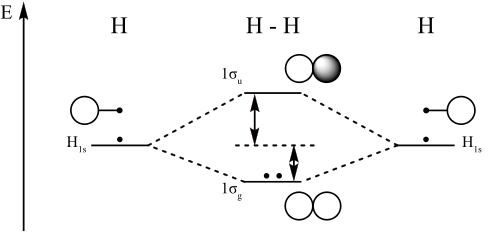## Linear Combination of Atomic Orbitals (LCAO)

#### Learning Objective

• Predict which orbitals can mix to form a molecular orbital based on orbital symmetry, and how many molecular orbitals will be produced from the interaction of one or more atomic orbitals

#### Key Points

• Electron configurations of atoms are described as wave functions.
• Wave functions are the basic set of functions that describe a given atom’s electrons.
• In chemical reactions, orbital wave functions are modified—the electron cloud shape is changed—according to the type of atoms participating in the chemical bond.
• The basis functions are one-electron functions centered on the nuclei of component atoms in a molecule.
• Minimizing the total energy of the system determines an appropriate set of linear combinations’ coefficients.
• The shape of the molecular orbitals and their respective energies are approximated by comparing the energies of the individual atoms’ atomic orbitals—or molecular fragments—and applying known values for level repulsion and other similar factors.

#### Terms

• diatomicconsisting of two atoms
• orbitala specification of an electron’s energy and probability density at any point in an atom or molecule
• wavefunctiona mathematical function describing the propagation of the quantum mechanical wave associated with a particle (or system of particles), related to the probability of finding the particle in a particular region of space

An atomic orbital is a mathematical function that describes the wave-like behavior of either one electron or a pair of electrons in an atom. This function can be used to calculate the probability of finding any electron in any specific region around an atom’s nucleus. An orbital may also refer to the physical region where the electron can be calculated to exist, as defined by the orbital’s particular mathematical form.

## Describing Molecules

Molecules are built from two or more bound atoms. It is possible to combine the known orbitals of constituent atoms in a molecule to describe its electron orbitals. Molecular orbitals (MOs) represent regions in a molecule where an electron is likely to be found; they are obtained by combining atomic orbitals. An MO can specify a molecule’s electron configuration, and most commonly, it is represented as a linear combination of atomic orbitals (the LCAO-MO method), especially in qualitative or approximate usage. These models provide a simple model of molecule bonding, understood through molecular orbital theory.Molecular orbital diagram for hydrogenFor a diatomic molecule, an MO diagram effectively shows the energetics of the bond between the two atoms, whose AO unbonded energies are shown on the sides. The unbonded energy levels are higher than those of the bound molecule, which is the energetically-favored configuration.

## Linear Combination of Atomic Orbitals

A linear combination of atomic orbitals, or LCAO, is a quantum superposition of atomic orbitals and a technique for calculating molecular orbitals in quantum chemistry. In quantum mechanics, electron configurations of atoms are described as wave functions. In a mathematical sense, these wave functions are the basic functions that describe the a given atom’s electrons. Orbital wave functions are modified in chemical reactions—the electron cloud shape changes—according to the type of atoms participating in the chemical bond.

One of LCAO’s initial assumptions is that the number of molecular orbitals is equal to the number of atomic orbitals included in the linear expansion. Essentially, n atomic orbitals combine to form n molecular orbitals.

Molecular orbital diagrams are diagrams of MO energy levels, shown as short horizontal lines in the center. Atomic orbitals (AO) energy levels are shown for comparison. Lines, often dashed diagonal lines, connect MO levels with their constituent AO levels. Levels with the same energy are commonly shown side by side. Appropriate AO and MO levels are filled with electrons symbolized by small vertical arrows, whose directions indicate the electron spins.